## 1.3.3 Modification of the numbers

Taking the role of the sub-domain and reference numbers into account, it could be useful to change some numbers during processing. For example, when a domain exhibits some symmetries or repetitions, a primal mesh is modified by these transformations. In this case, a number given can be introduced which changes value in order to be able to define different physical conditions. For example:

Sub-domain number 1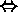Sub-domain number 1 (unchanged)

Sub-domain number 2Sub-domain number 3 (changed)

Reference number 1reference number 2 (changed)

Reference number 2reference number 3 (changed)

The modification modules perform this type of operation.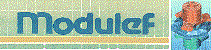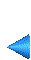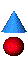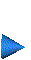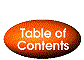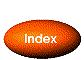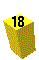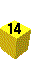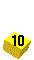Next: 1.3.4 Definition of the nodes Up: 1.3 Main ideas Prev: 1.3.2 Introduction of a curve Index Contents